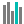#NORM.S.DIST (PQL - xl)

This function returns the standard normal cumulative distribution function

• Library: PQL \ Spreadsheet \ Statistical
• Compatibility: Any content (regardless of data source) in the Tabulate spreadsheet module

#### Syntax

NORM.S.DIST(z, cumulative)

##### Function Arguments
 Name Description Type Optional z A numeric value for which you want the distribution Number cumulative A logical value that determines the form of the function. If cumulative is TRUE, the function returns the cumulative distribution function; if FALSE, it returns the probability mass function StringOrNumber